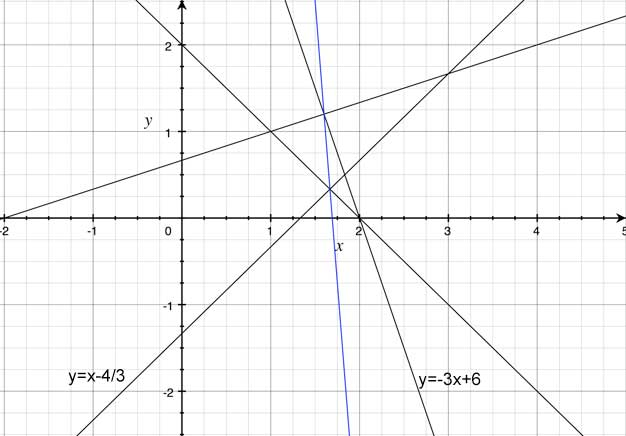SEARCH HOMEMath Central Quandaries & QueriesQuestion from mukulu, a student: Please help I've been trying to search in your data but i failed to get the solution Find the equation of a straight line joining the feet of the perpendiculars drawn from the point A(1,1) to the line 3x-3y-4= 0 and 3x+y-6=0.Hi Mukulu,

First find each of the perpendicular lines that pass through the point (1,1). The given lines and their corresponding perpendicular lines will intersect at a point which can be found using some simple algebra. These intersection points are the perpendicular feet. Once you have two points of a line, you can find the equation of the line that passes through them.

I included the graph of all of the lines involved in the question. The given equations are labeled (written in slope-intercept form) and the answer is in blue.Hope this helps. Write back if you still can't get the answer.

JaniceMath Central is supported by the University of Regina and The Pacific Institute for the Mathematical Sciences.